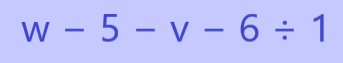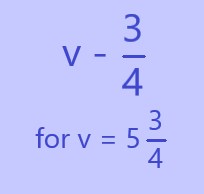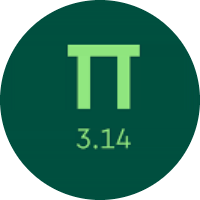# Understanding the Variable Expressions

In this quiz, you will get to know about the variable expressions. You will learn about writing the variable expressions, evaluating the expressions, identifying terms, and many more. You will also learn about the properties of addition and multiplication through these variable expressions.

Start Quiz

Write an expression for the operation described below.

multiply 6 by q

6.q

6/q

Write an expression for the sequence of operations described below.

divide 4 by 2, then subtract z from the result

Do not simplify any part of the expression.

4/2 - z

4.2 - z

2/4 - z

Sam had 65 caramels. Then, Sam’s sister took c of the caramels. Choose the expression that shows the number of caramels Sam has left.

c

65 - c

c - 65

Find the value of the expressionfor v = 3 and w = 16.

2

6

8

Find the value of the expression5

4

3

Lara works at a party supply store. Today, she is filling balloons with helium for the store to sell. She accidentally overfilled the first few balloons and they popped! If t is the total number of balloons she filled, then t–3 is the number of balloons that can be sold. Luna filled 41 balloons today during her time at work.

How many of the balloons Lara filled today can be sold?

41

38

How many terms are in this expression?

2k + 4n + 5

2

3

1

Which expression is equivalent to h+h+h+1+1+1?

3h + 3

3h + 2

4h + 3

Which property of addition is shown?

k + m = m + k

associative

commutative

identity

Which property of multiplication is shown?

m · 0 = 0

associative

identity

zero

Which expression shows a way to factor 26 + 44?

2(13 + 22)

2(13 + 24)

4(6 + 11)

Combine like terms in the expression.

8m - 2m + 5m + m

12m

11m

14m

Quiz/Test Summary
Title: Understanding the Variable Expressions
Questions: 12
Contributed by: